# Fractions Class 6 Maths Notes - Chapter 7

A fraction is defined as a part of a whole number. It can be expressed as a ratio between two integers separated by a solidus. The number in the upper part of a fraction is termed as numerator whereas the number in the lower part is termed as the denominator. For example, let us consider a fraction

$$\begin{array}{l}\frac{3}{12}\end{array}$$
where,
• 3 is the numerator
• 12 is the denominator
• It is read as three-twelfths

## Types of Fractions

Let us understand the different types of fractions. There are five types of fractions. They are proper fractions, improper fractions, mixed fractions, like fractions and unlike fractions.

• Proper fractions – It is a type of fraction where the denominator is always greater than the numerator. Some examples are
$$\begin{array}{l}\frac{4}{5}\end{array}$$
,
$$\begin{array}{l}\frac{3}{7}\end{array}$$
• Improper fractions – It is a type of fraction where the denominator is always less than the numerator. Some examples are
$$\begin{array}{l}\frac{5}{4}\end{array}$$
,
$$\begin{array}{l}\frac{7}{3}\end{array}$$
• Mixed fractions – It is a type of fraction which consists of a whole number and a proper fraction. Some examples are
$$\begin{array}{l}16\frac{3}{7}\end{array}$$
,
$$\begin{array}{l}3\frac{4}{5}\end{array}$$
• Like fractions – The type of fractions which have same denominators are called, like fractions. Some examples are
$$\begin{array}{l}\frac{1}{15}\end{array}$$
,
$$\begin{array}{l}\frac{3}{15}\end{array}$$
• Unlike fractions – The type of fractions which have different denominators are called, unlike fractions. Some examples are
$$\begin{array}{l}\frac{6}{27}\end{array}$$
,
$$\begin{array}{l}\frac{6}{28}\end{array}$$

#### For more information on Types of Fractions, watch the below videos## Introduction to Fractions

• A fraction is a number that represents a part of a whole.
• The whole may be a single object or a group of objects.
• Fraction=Numerator/Denominator
• Example: 1/2, 3/7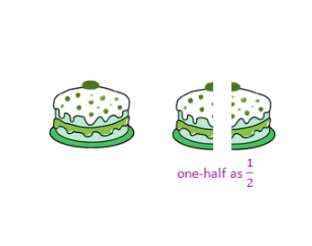### Representing Fractions

• Fractions can be represented using numbers, figures or words.

​​​​​​​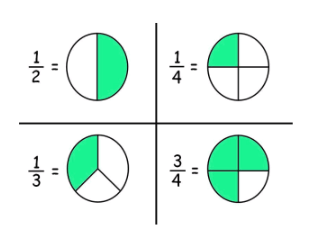​​​​​​​To know more about Fractions, visit here.

## Avatars of Fractions

### Proper Fractions

• If numerator < denominator in a fraction, then it is a proper fraction.
• Example: 2/3 and 4/9

### Improper and Mixed Fraction

• If numerator > denominator in a fraction, then it is an improper fraction.
• Example: 4/3 and 8/11
• An improper fraction can be written as a combination of a whole and a part, and is called mixed fractions.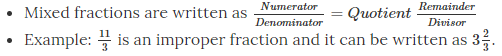To know more about Mixed Fractions, visit here.

### Interconversion between Improper and Mixed Fractions

Improper to mixed fraction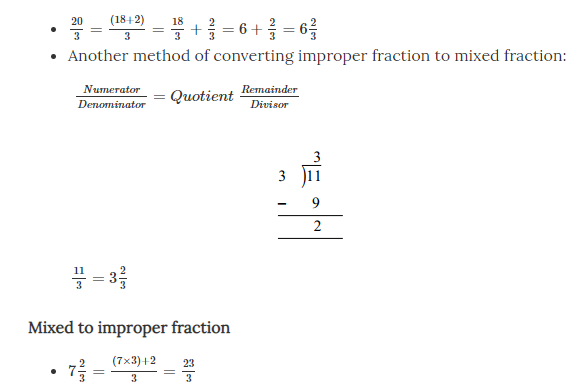## Meet the Twin Fractions

### Equivalent Fractions

• Each proper or improper fraction has many equivalent fractions.
• Multiply both numerator and denominator by a number, to find an equivalent fraction for the fraction.

Example: 1/2 and 2/4 are equivalent fractions.

• 1/3 + 7/3 = (1+7)/3 = 8/3
• 1/3 + 2/4 = (4+6)/12 = 10/12 = 5/6

### LCM

• Least common multiple of two numbers (LCM) is the smallest number that gets divided by both the numbers.
• Example: LCM of 3 and 4 is 12.## Let’s Subtract Fractions

### Subtraction of Fractions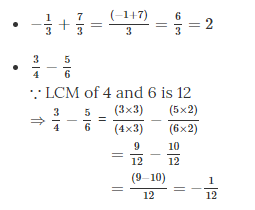## Many Many Many Fractions Together

### Multiplication of Fractions

Proper fraction * Proper fraction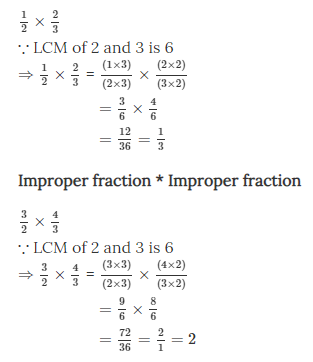Proper fraction * Improper fraction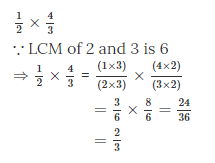To know more about Multiplication of Fractions, visit here.

## Let’s Divide Fractions

### Reciprocals of Fractions

• Turning the fraction upside down gives the Reciprocal of a fraction.
• Fraction × (Reciprocal of the fraction) = 1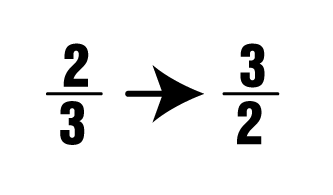To know more about Reciprocal of Fractions, visit here.

### Division of Fractions

• 1/2 ÷ 1/3
1/2 × Reciprocal of (1/3)
=1/2 × 3 = (1×3)/2
=3/2
• 4/3 ÷ 3/2
=4/3 × Reciprocal of (3/2)
=4/3×2/3=8/9

To know more about Division of Fractions, visit here.

### Comparison of Fractions

• Comparing like fractions with same denominators

2/3 and 8/3
2 < 8
∴ 2/3 < 8/3

• Comparing unlike fractions with same numerators

1/3 and 1/4
Portion of the whole showing 1/3 > Portion of the whole showing 1/4
∴1/3>1/4

• Comparing unlike fractions with different numerators

5/6 and 13/15
LCM of 6 and 15: 30
(5×5)/(6×5) = 25/30
(13×2)/(15×2)=26/30
⇒ 25/30<26/30
∴ 5/6<13/15

### Fractions on the Number Line

• The following figure shows how fractions 14, 24 and 34 are represented on a number line.
• Divide the portion from 0 to 1 on the number line into four parts.
• Then each part represents 1/4th portion of the whole.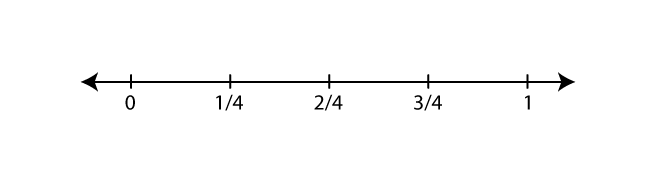Whole Numbers Integers

## Frequently Asked Questions on CBSE Class 6 Maths Notes Chapter 7 Fractions

Q1

### What are the different types of fractions?

The different types of fractions are 1. Proper Fractions 2. Improper Fractions 3. Mixed Fractions 4. Like Fractions 5. Unlike Fractions 6. Equivalent Fractions 7. Unit Fractions

Q2

### What is a mixed fraction?

A mixed fraction is defined as a fraction formed by combining a whole number and a fraction.

Q3

### What is ‘LCM’?

LCM is least common multiple. For two integers a and b, the LCM is the smallest positive integer that is evenly divisible by both a and b.